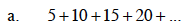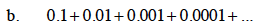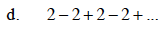### Home > CALC > Chapter 10 > Lesson 10.1.2 > Problem10-9

10-9.
1. For each of the following series, decide if there is a finite sum. Then predict the sum. If there is not a finite sum, explain why. Homework Help ✎

1. 5 + 10 + 15 + 20 + …

2. 0.1 + 0.01 + 0.001 + 0.0001 + …

3.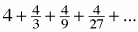4. 2 − 2 + 2 − 2 + …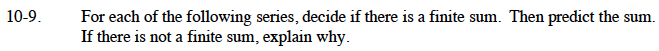For an infinite geometric series:

But r must be _____.

The series in part (a) is arithmetic.
The other series are geometric. What is the value of r in each case?